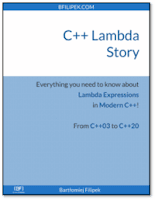# 5 Curious C++ Lambda Examples: Recursion, constexpr, Containers and More

Please have a look at my quick blog post where I’ll show you a few interesting lambda examples. Do you know how to write a recursive lambda? Store them in a container? Or invoke at compile time?

See in the article

## 1. Recursive Lambda with `std::function`

Writing a recursive function is relatively straightforward: inside a function definition, you can call the same function by its name. How about lambdas?

``````int main() {
auto factorial = [](int n) {
return n > 1 ? n * factorial(n - 1) : 1;
};
return factorial(5);
}``````

This, unfortunately, doesn’t compile…

How can we fix this?

One way is to use `std::function`:

``````#include <functional>

int main() {
const std::function<int(int)> factorial = [&factorial](int n) {
return n > 1 ? n * factorial(n - 1) : 1;
};
return factorial(5);
}``````

This time we need to capture `factorial` and then we can refer to it inside the lambda body.

And since C++14 we can also leverage generic lambdas and write the following code:

``````int main() {
const auto factorial = [](int n) {
const auto fact_impl = [](int n, const auto& impl) -> int {
return n > 1 ? n * impl(n - 1, impl) : 1;
};
return fact_impl(n, fact_impl);
};
return factorial(5);
}``````

This time it’s even more complicated (but doesn’t require heavy use of `std::function`). It uses internal lambda for the main computation and then it’s passed as a generic argument.

But I wonder: have you ever used recursive lambdas? Or it’s better to rely on recursive functions (which seems to be far more comfortable to use and write).

## 2. `constexpr` Lambdas

But that’s not all with recursion… :)

Since C++17 we can write lambdas that have the call operator defined as `constexpr`. We can use this property and expand the recursive example into:

``````int main() {
constexpr auto factorial = [](int n) {
constexpr auto fact_impl = [](int n, const auto& impl) -> int {
return n > 1 ? n * impl(n - 1, impl) : 1;
};
return fact_impl(n, fact_impl);
};
static_assert(factorial(5) == 120);
}``````

And in C++20 you can even apply `consteval` to mark lambdas which can be evaluated only at compile time.

## 3. Storing Lambdas in a Container

This might be a bit cheating… but we can theoretically store lambdas in a container.

While closure types have default constructors deleted (unless it’s stateless lambda in C++20), we can do a little hack and store all lambdas as `std::function` objects. For example:

``````#include <functional>
#include <iostream>
#include <vector>

int main() {
std::vector<std::function<std::string(const std::string&)>> vecFilters;

vecFilters.emplace_back([](const std::string& x) {
return x + " Amazing";
});
vecFilters.emplace_back([](const std::string& x) {
return x + " Modern";
});
vecFilters.emplace_back([](const std::string& x) {
return x + " C++";
});
vecFilters.emplace_back([](const std::string& x) {
return x + " World!";
});

const std::string str = "Hello";
auto temp = str;

for (auto &entryFunc : vecFilters)
temp = entryFunc(temp);

std::cout << temp;
}``````

## 4.Generic lambdas and Help with Deduction

C++14 brought an important addition to lambdas: generic lambda arguments. Here’s one example that shows why is it useful:

``````#include <algorithm>
#include <iostream>
#include <map>
#include <string>

int main() {
const std::map<std::string, int> numbers {
{ "one", 1 }, {"two", 2 }, { "three", 3 }
};

std::for_each(std::begin(numbers), std::end(numbers),
[](const std::pair<std::string, int>& entry) {
std::cout << entry.first << " = " << entry.second << '\n';
}
);
}``````

Do you know what’s the mistake here? Is the argument type appropriately specified in the inner lambda for `for_each`?

I specified: `const std::pair<std::string, int>& entry`.

But it’s wrong as the type of the key/value pair inside a map is:

`std::pair<const std::string, int>`

That’s why the compiler has to create unwanted temporary copies and then pass them to my lambda.

We can quickly fix this by using a generic lambda from C++14.

``````std::for_each(std::begin(numbers), std::end(numbers),
[](const auto& entry) {
std::cout << entry.first << " = " << entry.second << '\n';
}
);``````

Now the types match, and no additional copies are created.

## 5. Returning a lambda

If you want to return a lambda from a function (for example for partial function application, currying), then it’s not straightforward because you don’t know the exact type of the closure object.

In C++11 one way was to use `std::function`:

``````#include <functional>

std::function<int(int)> CreateLambda(int y) {
return [y](int x) { return x + y; };
}

int main() {
auto lam = CreateLambda(10);
return lam(32);
}``````

But since C++14, we can leverage the auto type deduction for return types and just write:

``````auto CreateLambda(int y) {
return [y](int x) { return x + y; };
}

int main() {
auto lam = CreateLambda(10);
return lam(32);
}``````

The above code is far simpler and cheaper as we don’t need to use `std::function`.

## Summary

In this quick article, I showed you five interesting lambda examples. They might not be common, but shows flexibility and sometimes even complexity of the closure types.

Do you use lambdas in such contexts?
Or maybe you have even more complicated examples?C++ Lambda Story@Leanpub